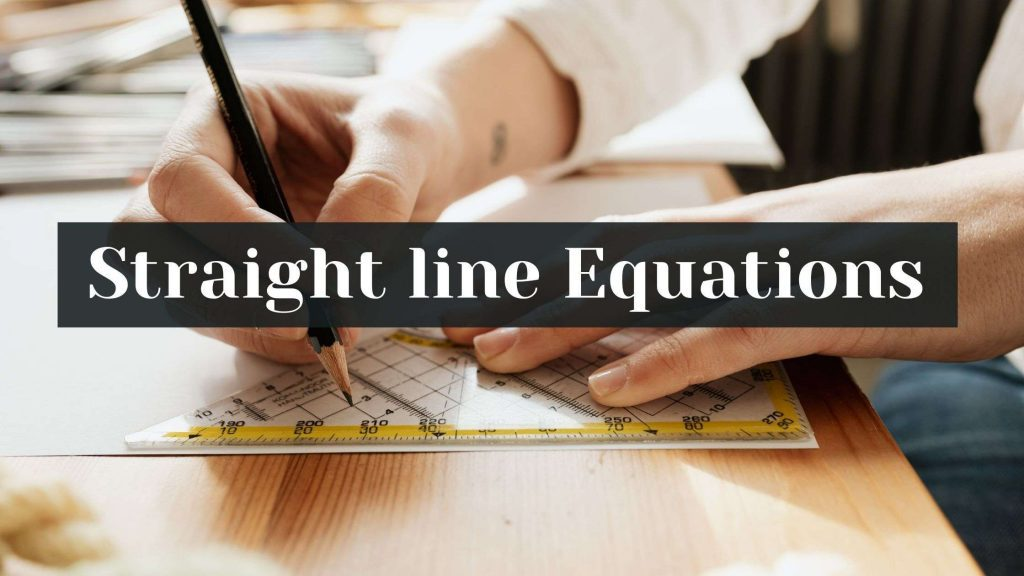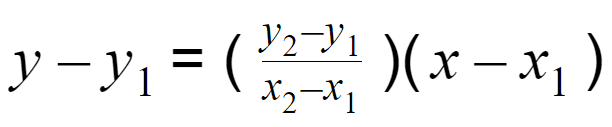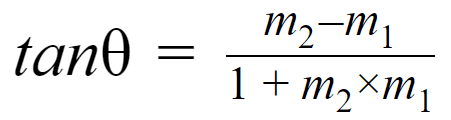Maths
Study Material

# Equation of a Straight Line | Different Forms |

A straight line is basically just a line with no curves. Usually, when we speak about lines, we are talking about straight lines. Furthermore, here we are going to learn about different forms of equation of straight line.

4 minutes longHesap Oluştur

Got stuck on homework? Get your step-by-step solutions from real tutors in minutes! 24/7. Unlimited.

A straight line is basically just a line with no curves. Usually, when we speak about lines, we are talking about straight lines. Furthermore, here we are going to learn about different forms of equation of a straight line.

## Straight Line – Definition

According to the definition, a straight line is the set of all points between and extending beyond two points.
Furthermore, the main properties of straight lines are that they have only one dimension, length, and they extend in two directions forever.

## Slope of a line

The slope(gradient) of a line is a number that represents both the direction and the inclination of the line. It is computed as the difference in y coordinates/difference in x coordinates, which is also called rise over run. Generally, It is denoted by m.

Also, the value of slope can be determined as, m = tanθ where θ is the angle formed by the line with the positive x-axis.

## Equation of a Straight Line

A straight line equation is a linear equation. On a Cartesian plane it can have different representations based on the given angles, variables, and constants, below are the different forms of straight lines.

## General Equation of a Straight Line

• General equation of a straight line is represented as ax + by + c = 0, where
• a, b, c are constants, and
• x, y are variables.
• The slope is -a/b

## Slope-Intercept Form

• The slope-intercept is the most popular form of equation of a straight line. Many find this useful because of its simplicity. Further, It is represented as:
• y = mx + b
• where m is the slope and
• b is the y-intercept

## Point Slope Form

• In case of point slope form, it is derived from slope-intercept form. This equation of a straight line is used when the slope and any one point A(x₁,y₁) is given, represented as:
• y-y₁ = m(x-x₁)

## Two Point Form

• This form of equation of a straight line is used when there is two known points A(x₁,y₁) and B(x₂,y₂) and we need to find the equation of the line passing through these two points.
• Further, it is represented as:## Intercept Form

• Generally, a straight line having x-intercept as a and y-intercept as b as shown in the figure where point A is on the x-axis and point B is on the y-axis, is given in the intercept form by:
• x/a + y/b = 1

## Angle between two Lines

• The acute angle θ between two lines having slopes m₁ and m₂, where m₂>m₁ can be determined using the formula:## Picked For You

Furthermore, these are some topics which might interest you: